How to Learn in 24 Hours?

 Need Help? M-F: 9am-5pm(PST): Toll-Free: (877) RAPID-10 or 1-877-727-4310 24/7 Online Technical Support: The Rapid Support Center vip@rapidlearningcenter.com Secure Online Order:Need Proof? Testimonials by Our Users

 Rapid Learning Courses: MCAT in 24 Hours (2021-22) USMLE in 24 Hours (Boards) Chemistry in 24 Hours Biology in 24 Hours Physics in 24 Hours Mathematics in 24 Hours Psychology in 24 Hours SAT in 24 Hours ACT in 24 Hours AP in 24 Hours CLEP in 24 Hours DAT in 24 Hours (Dental) OAT in 24 Hours (Optometry) PCAT in 24 Hours (Pharmacy) Nursing Entrance Exams Certification in 24 Hours eBook - Survival Kits Audiobooks (MP3)

 Tell-A-Friend: Have friends taking science and math courses too? Tell them about our rapid learning system.Home »  Mathematics »  High School Algebra 2

Using and Verifying Identities

 Topic Review on "Title": Reciprocal identities and quotient identities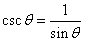,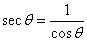,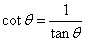,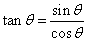and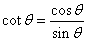. Pythagorean identities: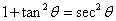,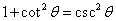, and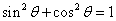.  Co-function identities: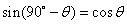,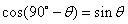and. Sum and difference identities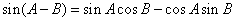,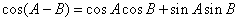and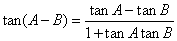;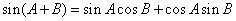,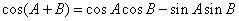and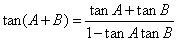Double angle identities: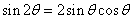,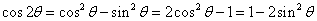and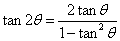. Power reducing and half angle formulas: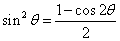,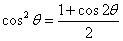and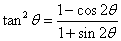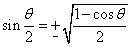,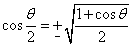and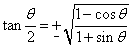Sum-to-product formulas: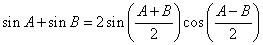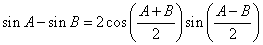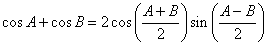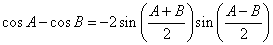Product-to sum formulas:Rapid Study Kit for "Title":
 Flash Movie Flash Game Flash Card Core Concept Tutorial Problem Solving Drill Review Cheat Sheet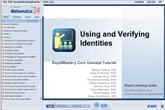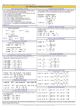"Title" Tutorial Summary : This tutorial introduces the known trigonometric identities and their use in the simplification process of trigonometric expressions.  Known trigonometric identities helped reduce the time it takes to simplify trigonometric expressions. The fundamental trigonometric functions are shown in the examples provided with relation to specific scenarios. The Pythagorean identities are derived with the knowledge of one of them. Difference and sum identities of the sine, cosine and tangent functions are shown in this tutorial. They come handy when simplification of trig expressions is needed.

 Tutorial Features: Specific Tutorial Features: • The various trigonometric formulas are shown in the examples. • Step by step examples are shown to verify trigonometric identities. Series Features: • Concept map showing inter-connections of new concepts in this tutorial and those previously introduced. • Definition slides introduce terms as they are needed. • Visual representation of concepts • Animated examples—worked out step by step • A concise summary is given at the conclusion of the tutorial.

 "Title" Topic List: Using and verifying identities Fundamental trigonometric functions Pythagorean identities Simplifying expressions Periodicity of trigonometric identities Co-function identities Difference identities Sum identities Double angle identities Proof of double angle sine identities Proof of double angle cosine identities Negative angle formulas Proof of sine negative angle formulas Proof of cosine negative angle formulas Power reducing formulas Half angle identities Sum-to-product formulas Product-to-Sum formulas

See all 24 lessons in High School Algebra 2, including concept tutorials, problem drills and cheat sheets:
Teach Yourself High School Algebra 2 Visually in 24 Hours